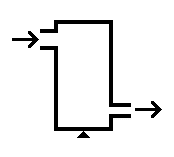#### You may also like### Mach Attack

Have you got the Mach knack? Discover the mathematics behind exceeding the sound barrier.### Moving Stonehenge

A look at the fluid mechanics questions that are raised by the Stonehenge 'bluestones'.

##### Age 16 to 18Challenge Level

You may have learned Newton's 2nd law of motion, "force is equal to the rate of change of momentum". In fluids, the rate of change of mass, $dm/dt$, often abbreviated $\dot{m}$, is important. Use the product rule to find the form of Newton 2 that includes the possibility of mass flow.

If the flow is "steady", i.e. the mass flow in to a certain volume equals the mass flow out, the formula you derived simplifies to $F = \dot{m}v$.

When we resolve this formula in any particular direction, we call it the "steady flow momentum equation".

A tank (pictured) has a chemical (density $800kg/m^3$) flow of 1kg/s going through from left to right. The inlet pipe has an area $100cm^2$, and the outlet pipe has an area $50cm^2$. If the inlet pressure is 1MPa, what is the output pressure? Hint: mass is conserved, and the mass flow in a pipe of area A with fluid velocity V is just $\dot{m} = \rho AV$.Can you see any structural problems that might arise with this tank?

Would it make a significant difference if the tank were aligned vertically or horizontally?